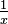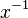# Reciprocal facts for kids

Kids Encyclopedia Facts

In mathematics, the reciprocal (or multiplicative inverse) of a number is 1 divided by the number, or equivalently, the number raised to the power of -1 (as in$\tfrac{1}{x}$ and$x^{-1}$). All numbers have a reciprocal except zero, since no number times 0 is 1. Two numbers are reciprocal of each other if and only if their product is 1. For example:

• 2.5 and 0.4 are reciprocals, because 2.5 × 0.4 = 1.
• -0.2 and -5 are reciprocals, because -0.2 × -5 = 1.
• 1 and -1 are their own reciprocals, because 1 × 1 = 1 and -1 × -1 = 1.

To find the reciprocal of a fraction, swap the numerator and the denominator. Whole numbers can be thought as having a denominator of 1. For example:

• The reciprocal of 8 is 1/8 (or 0.125).
• The reciprocal of 5/3 is 3/5 (or 0.6).
• The reciprocal of 1/7 is 7.
• The reciprocal of -9/4 is -4/9.

Dividing a fraction is the same as multiplying its reciprocal and vice versa.

## Related pagesReciprocal Facts for Kids. Kiddle Encyclopedia.